## Tamilnadu Samacheer Kalvi 9th Maths Solutions Chapter 8 Statistics Additional Questions

Exercise 8.1

Question 1.
The following data gives the number of residents in an area based on their age. Find the average age of the residents.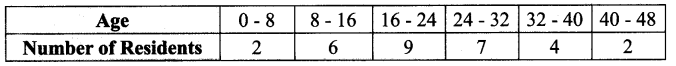Solution: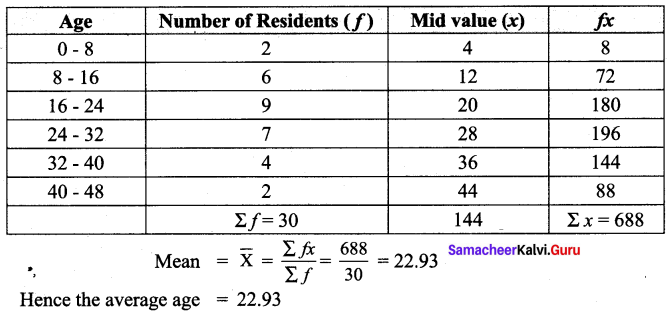Question 2.
Find the mean for the following frequency table :Solution:
Let Assumed mean A = 170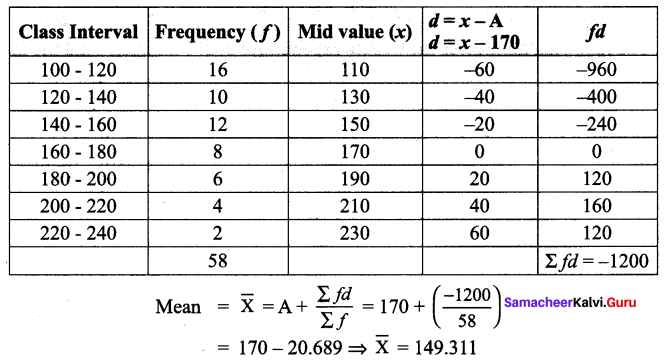Question 3.
Find the mean for the following distribution using step Deviation Method.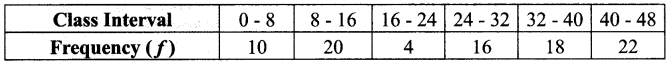Solution:
Let Assumed mean A = 28, Class width C = 8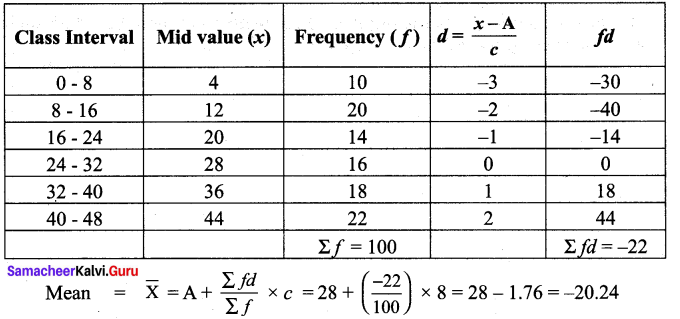Exercise 8.2

Question 1.
For the following up grouped data 8, 15, 14, 19, 11, 16, 10, 8, 17, 20. Find the median.
Solution:
Arrange the values in ascending order 8, 8, 10, 11, 14, 15, 16, 17, 19, 20
The number of values = 10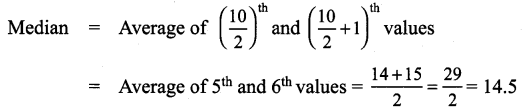Question 2.
The following table gives the weekly expenditure of 200 families. Find the median of the weekly expenditure.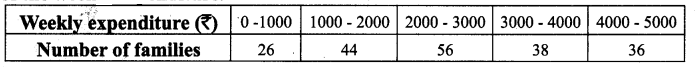Solution: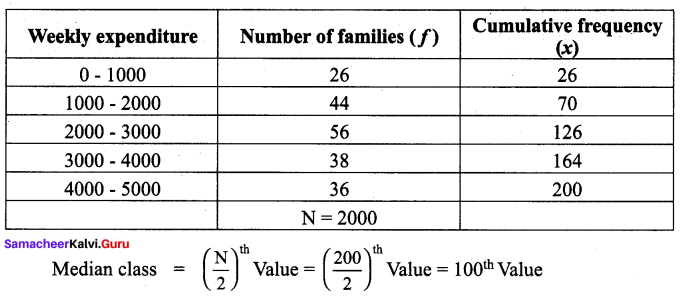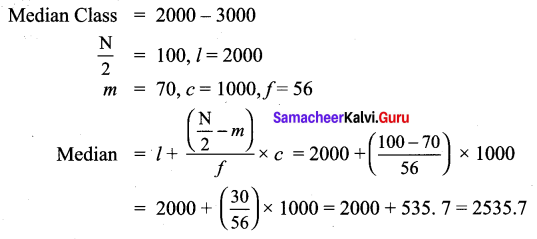Question 3.
The median of the following data is 24. Find the value of x.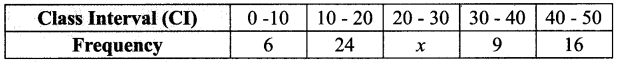Solution: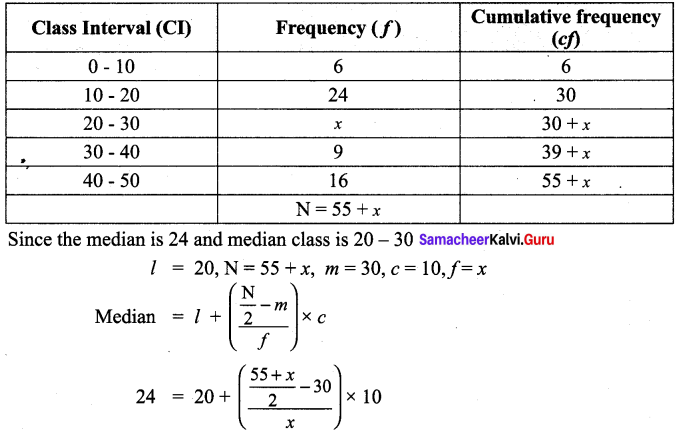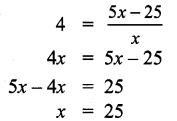Question 4.
The following are the scores obtained by 11 players in a cricket match 7, 21, 45, 12, 56, 35, 25, 0, 58, 66, 29. Find the median score.
Solution:
Let us arrange the values in ascending order 0, 7, 12, 21, 25, 29, 35, 45, 56, 58, 66
The number of values = 11 which is odd.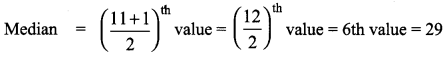Exercise 8.3Question 1.
Find the mode of the given data : 65, 65, 71, 71, 72, 75, 82, 72, 47, 72.
Solution:
In the given data 72 occurs thrice. Hence the mode is 72.

Question 2.
Find the mode: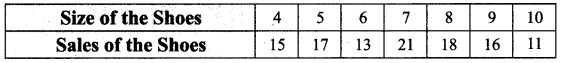Solution:
7 has the maximum frequency 21. Therefore 7 is the mode.

Question 3.
Find the mode for the following data.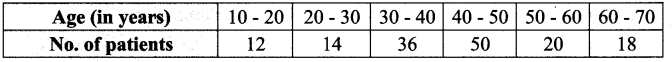Solution: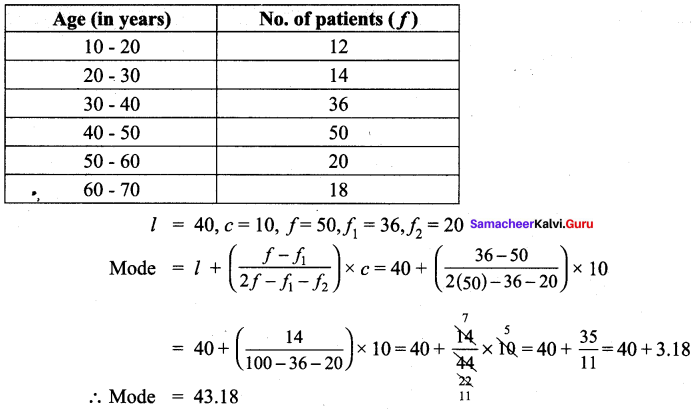Question 4.
In a distribution, the mean and mode are 46 and 40 respectively. Calculate the median.
Solution:
Given, Mean = 46 and mode = 40
Using mode ≈ 3 median – 2 mean ,
40 ≈ 3 Median – 2 (46)
3 Median ≈ 40 + 92
Therefore, Median ≈ $$\frac{132}{3}$$ = 44

Exercise 8.4Multiple Choice Questions :

Question 1.
The mean of first 10 natural numbers.
(1) 25
(2) 55
(3) 5.5
(4) 2.5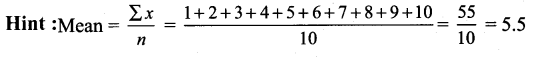Solution:
(3) 5.5

Question 2.
The mean of a distribution is 23, the median is 24 and the mode is 25.5. It is most likely that this distribution is :
(1) Positively skewed
(2) Symmetrical
(3) Asymptotic
(4) Negatively skewed
Hint: For Negatively skewed means is likely to be less than mode and median
Solution:
(4) Negatively skewed

Question 3.
The middle value of an ordered array of numbers is the
(1) Mode
(2) Mean
(3) Median
(4) Mid point
Solution:
(3) Median

Question 4.
The weights of students in a school is a :
(1) Discrete variable
(2) Continuous variable
(3) Qualitative variable
(4) None of these
Solution:
(2) Continuous variableQuestion 5.
The first hand and unorganized form data is called
(1) Secondary data
(2) Organised data
(3) Primary data
(4) None of these
Solution:
(3) Primary data